Next: Flat Lifting Wings Up: Two-Dimensional Compressible Inviscid Flow Previous: Linearized Subsonic Flow

# Linearized Supersonic Flow

The aim of this section is to re-examine the problem of supersonic flow past a thin, two-dimensional airfoil using the small-perturbation theory developed in Section 15.12.

As before, the unperturbed flow is of uniform speed, directed parallel to the-axis, and the associated Mach number is. The perturbed flow, due to the presence of the airfoil, is governed by Equation (15.127), which can be written in the hyperbolic form (see Section 15.14)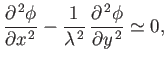(15.183)

where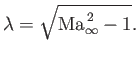(15.184)

As we saw in Section 15.14, the general solution of Equation (15.183) is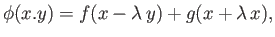(15.185)

whereand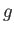are arbitrary functions. Because disturbances only propagate along downstream-running Mach lines (i.e., Mach lines that originate at the airfoil), we only need the functionfor the upper surface, and the functionfor the lower surface. Thus,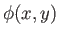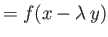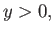(15.186)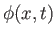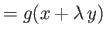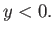(15.187)

On the upper surface, the boundary condition (15.129) yields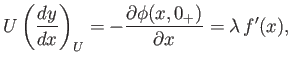(15.188)

which implies that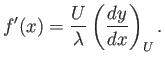(15.189)

Similarly,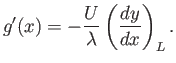(15.190)

According to Equation (15.128), the pressure coefficient on the surface of the airfoil is given by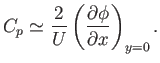(15.191)

Hence, we obtain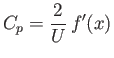(15.192)

on the upper surface, and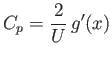(15.193)

on the lower surface. Thus,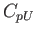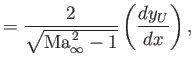(15.194)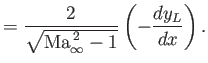(15.195)

This is the same result as that obtained in Section 15.9, where the pressure on thin airfoils was obtained by an approximation to the shock-expansion method. In fact, for the purposes of calculating the velocity and pressure perturbations on the surface of the airfoil, the linearized theory discussed in this section is equivalent to the weak wave approximations of Section 15.9.Next: Flat Lifting Wings Up: Two-Dimensional Compressible Inviscid Flow Previous: Linearized Subsonic Flow
Richard Fitzpatrick 2016-03-31